Next: Special case: White noise Up: Multi-variate Gaussian Integration: Previous: Case 2:

### Case 3: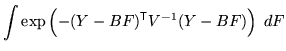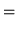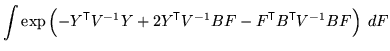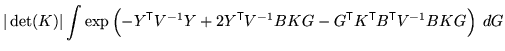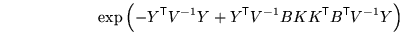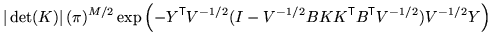where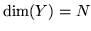,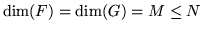,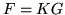and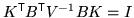. This assumes that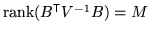so that.

For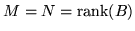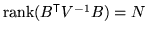implies that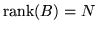and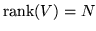. Consequently, using the SVD decompositions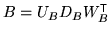and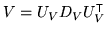gives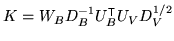. Therefore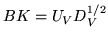and so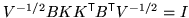, so that the integral is given by: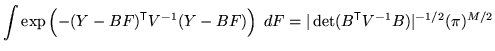(17)

For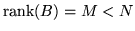,is taken to have full rank, but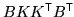is not, as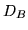is not a square matrix, so that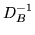does not exist. Instead,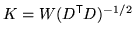where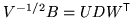by SVD decomposition. As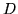has dimensions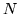by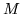(same as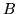) then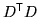isbyand hence invertible (by virtue ofbeing full rank). Therefore,as desired, but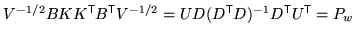which is a projection matrix. Consequently,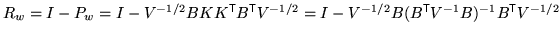is not zero, but is the residual projection matix (onto the null space of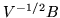- the prewhitened version of). The integral can then be written as: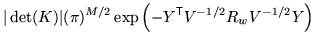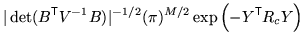(18)

where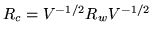is the residual projection matrix in the coloured space, and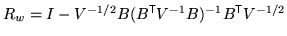is the residual projection matrix in the whitened space.

For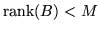,

In this case the matrixwill have many linearly dependent columns andcannot be achieved. Instead, let the number of independent columns be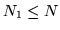. Furthermore, letby SVD, where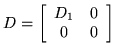where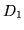is an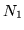bydiagonal matrix with all diagonal entries being non-zero. Integration of the parameters associated with the zero singular values can be carried out if they have finite extents. Letting, and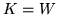gives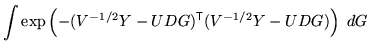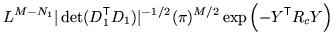(19)

where the null parameters are integrated over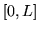, and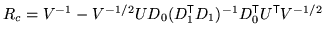, with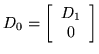which is anbymatrix.Next: Special case: White noise Up: Multi-variate Gaussian Integration: Previous: Case 2: